# Marginal effects vs Wald tests (Stata)

Calli Cain, a criminologist from FAU asks:

What is the best method to examine whether there are group differences (e.g., gender, race) in the effects of several variables on binary outcomes (using logistic regression)? For example – if you want to look at the gendered effects of different types of trauma experiences on subsequent adverse behaviors (e.g., whether participant uses drugs, alcohol, has mental health diagnosis, has attempted suicide). Allison (1999) cautions against using Equality of Coefficients tests to look at group differences between regression coefficients like we might with OLS regression. If you wanted to look at the differences between a lot of predictors (n= 16) on various outcomes (n=6) – what would be best way to go about it (I know using interaction terms would be good if you were just interested in say gender differences of one or two variables on the outcome). Someone recommended comparing marginal effects through average discrete changes (ADCs) or discrete changes at representative values (DCRs) – which is new to me. Would you agree with this suggestion?

When I am thinking about should I use method X or method Y type problems, I think about the specific value I want to estimate first, and then work backwards about the best method to use to get that estimate. So if we are talking about binary endpoints such as uses drugs (will go with binary for now, but my examples will readily extend to say counts or real valued outcomes), there are only generally two values people are interested in; say the change in probability is 5% given some input (absolute risk change, e.g. 10% to 5%), or a relative risk change such as X decreases the overall relative risk by 20% (e.g. 5% to 4%).

The former, absolute change in probabilities, is best accomplished via various marginal effects or average discrete changes as Calli says. Most CJ examples I am aware of I think these make the most sense to focus on, as they translate more directly to costs and benefits. Focusing on the ratio’s sometimes makes sense, such as in case-control studies, or if you want to extrapolate coefficient estimates to a very different sample. Or if you are hyper focused on theory and the underlying statistical model.

Will show an example in Stata using simulated data to illustrate the differences. Stata is very nice to work with different types of marginal estimates. Here I generate data with three covariates, female/males, and then some interactions. Note the covariate x1 has the same effect for males/females, x2 and x3 though have countervailing effects (females it decreases, males it increases the probability).

``````* Stata simulation to show differences in Wald vs Margins
clear
set more off
set seed 10
set obs 5000
generate female = rbinomial(1,0.5)
generate x1 = rnormal(0,1)
generate x2 = rnormal(0,1)
generate x3 = rnormal(0,1)

* x1 same effect, x2/x3 different across groups
generate logit = -0.1 + -2.8*female + 1.1*(x1 + x2 + x3) + -1.5*female*(x2 + x3)
generate prob = 1/(1 + exp(-1*logit))
generate y = rbinomial(1,prob)
drop logit prob``````

I intentionally generated the groups so females/males have quite different baseline probabilities for the outcome y here – something that happens in real victim data in criminology.

``````* Check out marginal differences
tabstat y, by(female)``````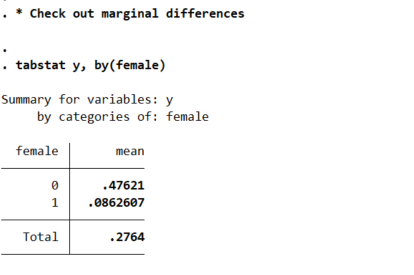So you can see males have the proportion of the outcome near 50% in the sample, whereas females are under 10%. So Calli is basically interested in the case below, where we estimate all pairwise interactions, so have many coefficient differences to test on the right hand side.

``````* Estimate model with interactions (linear coefficients)
logistic y i.female x1 x2 x3 i.female#(c.x1 c.x2 c.x3), coef``````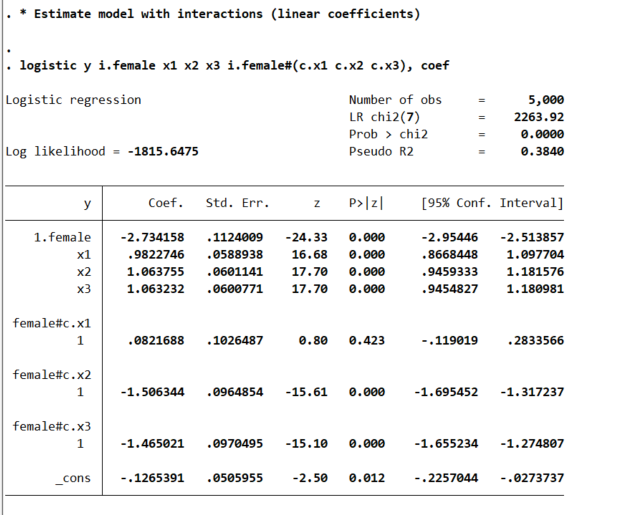This particular model does the Wald tests for the coefficient differences just by the way we have set up the model. So the interaction effects test for differences from the baseline model, so can see the interaction for x1 is not stat significant, but x2/x3 are (as they should be). But if you are interested in the marginal effects, one place to start is with derivatives directly, and Stata automatically for logit models spits out probabilities:

``````* Marginal effects
margins female, dydx(x1 x2 x3)
* x1 is the same linear effect, but margins are quite different``````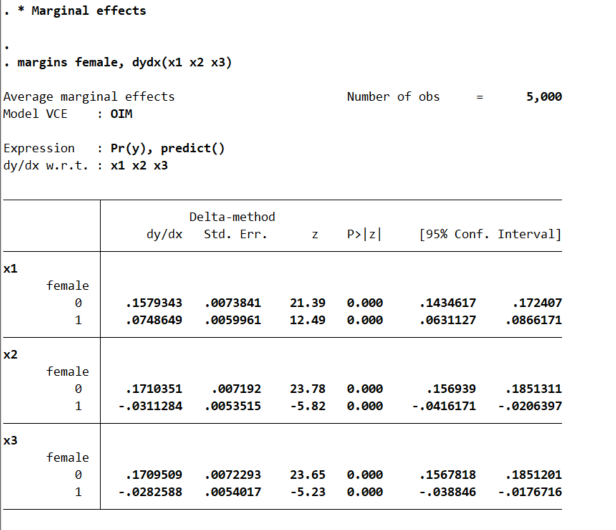So even though I made the underlying effect for x1 equal between males/females for the true underlying data generation process, you can see here the marginal derivative is much smaller for females. This is the main difference between Wald tests and margins.

This is ok though for many situations. Say x1 is a real valued treatment, such as Y is victimization in a sample of high risk youth, and x1 is hours given for a summer job. We want to know the returns of expanding the program – here the returns are higher for males than females due to the different baseline probabilities of risk between the two. This is true even if the relative effect of summer job hours is the same between the two groups.

Again Stata is very convenient, and we can test for the probability differences in males/females by appending `r.` to the front of the margins command.

``````* can test difference contrast in groups
margins r.female, dydx(x1 x2 x3)``````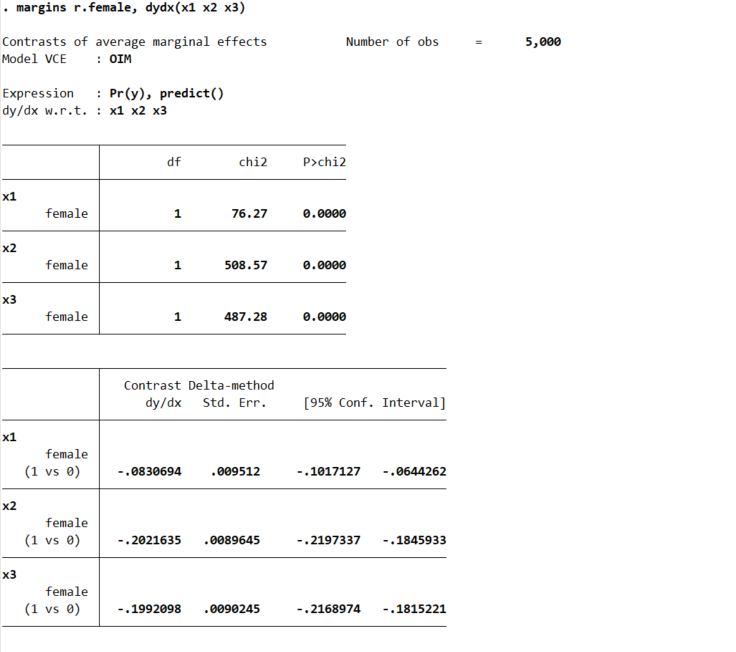But the marginal derivative can be difficult to interpret – it is the average slope, but what does that mean exactly? So I like evaluating at fixed points of the continuous variable. Going back to our summer job hours example, you could evaluate going from 0 to 50 hours, or going from 50 to 100, or 0 to 100, etc. and see the average returns in terms of reductions in the probability of trauma. Here because I simulated the data to be a standard normal, I just go from -1 to 0 to 1:

``````* Probably easier to understand at particular x1 values
margins female, at(x1=(-1(1)1))``````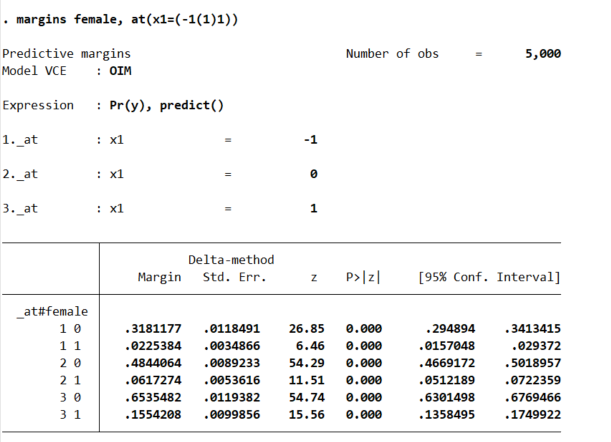So that table is dense, but it says when x1=-1, females have a probability of y of 2%, and males have a probability of y of 32%. Now go up the ladder to x1=0, females have a probability of 6% and males have a probability of 48%. So a discrete change of 4% for females and 16% for males. If we want to generate an interval around that discrete change effect:

``````* Can test increases one by one
margins female, at(x1=(-1 0 1))   contrast(atcontrast(ar) effects marginswithin)``````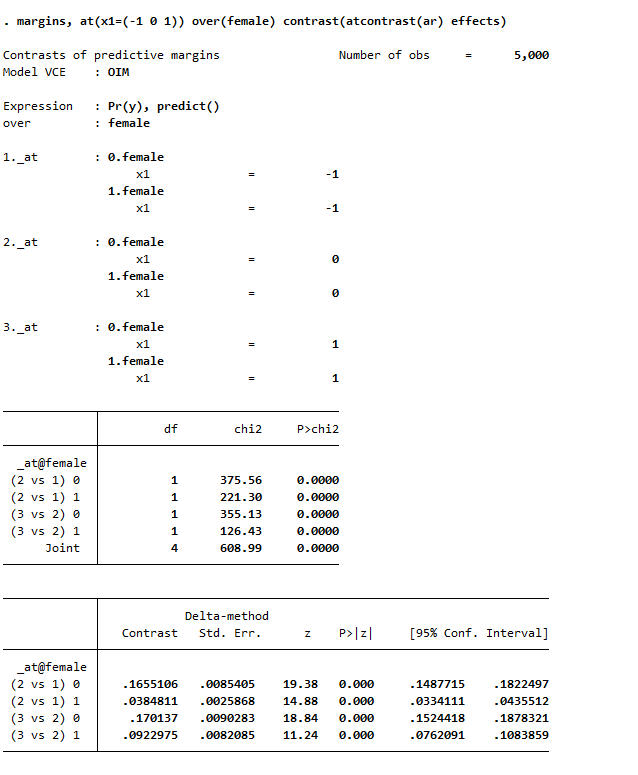See, isn’t Stata’s margins command so nice! (For above, it actually may make more sense to use `margins , at(x1=(-1 0 1)) over(female) contrast(atcontrast(ar) effects)`. Margins estimates the changes over the whole sample and averages filling in certain values, with over it only does the averaging within each group on over.) And finally we can test the difference in difference, to see if the discrete changes in males females from going from -1 to 0 to 1 are themselves significant:

``````* And can test increases of males vs females
margins r.female, at(x1=(-1(1)1)) contrast(atcontrast(ar))``````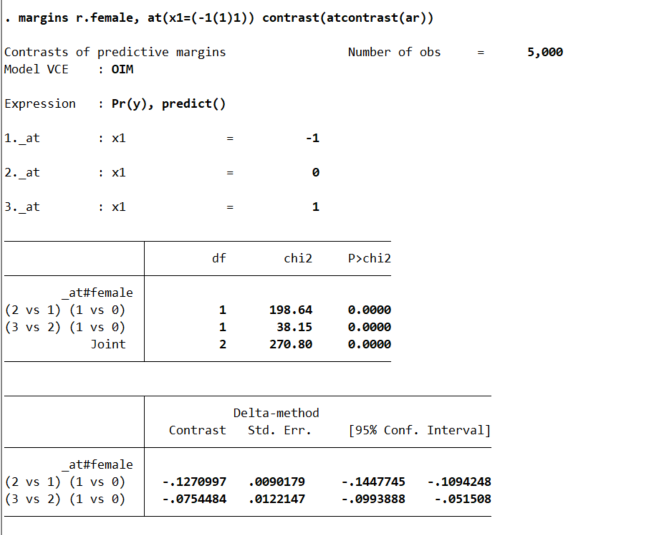So the increase in females is 13% points smaller than the increase in males going from -1 to 0, etc.

So I have spent alot of time on the probabilities so far. I find them much easier to interpret, and I do not care so much about the fact that it doesn’t necessarily say the underlying DGP is different from males/females. But many people are interested in the odds ratios (say in case-control studies). Or generalizing to a different sample, say this is a low risk sample of females, and you want to generalize the odds ratio’s to a higher risk sample with a baseline more around 50%. Then looking at the odds ratio may make more sense.

Or so far I have not even gotten to Calli’s main point, how to test many of these effects for no differences at once. There I would just suggest the likelihood ratio test (which does not have the problems with the Wald tests on the coefficients and that the variance estimates may be off):

``````* Estimate restricted model
logistic y ibn.female c.x1 c.x2 c.x3, coef noconstant
estimates store restrict

* Another way to do the full interaction model
* More like separate male and female
logistic y ibn.female ibn.female#(c.x1 c.x2 c.x3), coef noconstant
estimates store full

* LRT test between models
lrtest restrict full``````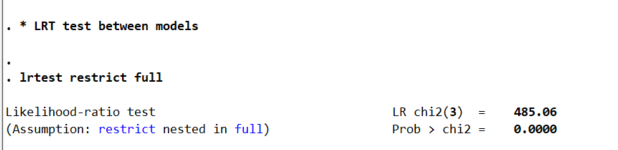So here as expected, one rejects the null that the restricted model is a better fit to the data. But this is pretty uninformative – I rather just go to the more general model and quantify the differences.

So if you really want to look at the odds ratios, we can do that using `lincom` post our full interaction model:

``logistic y i.female ibn.female#(c.x1 c.x2 c.x3), coef noconstant``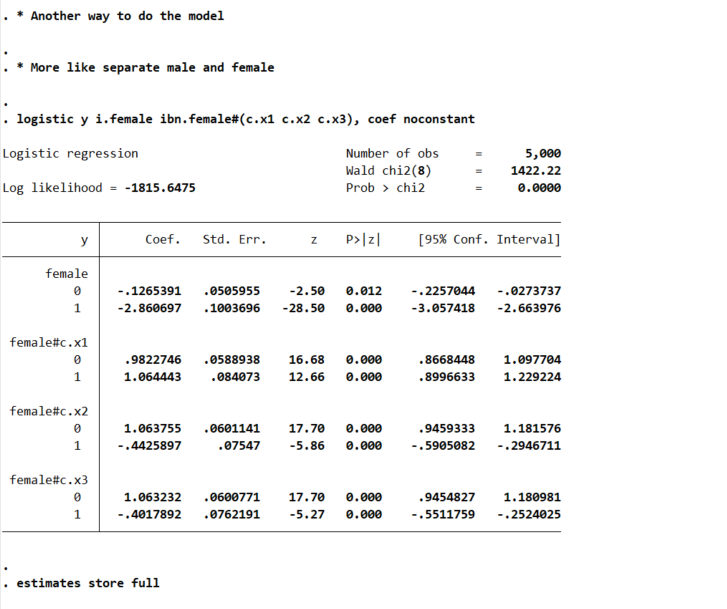And here is an example post Wald test for equality:

``lincom 0.female#c.x1 - 1.female#c.x1``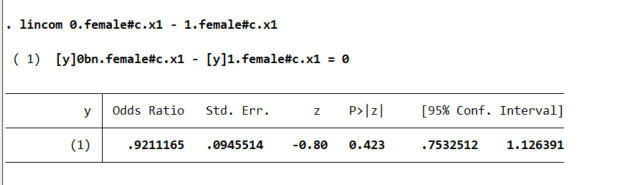You may ask where does this odd’s ratio of 0.921 come from? Well, way back in our first full model, the interaction term for `female*x1` is `0.0821688`, and `exp(-0.0821688)` equals that odds ratio and has the same p-value as the original model I showed. And so you can see that the x1 effect is the same across each group. But estimating the other contrasts is not:

``lincom 0.female#c.x2 - 1.female#c.x2``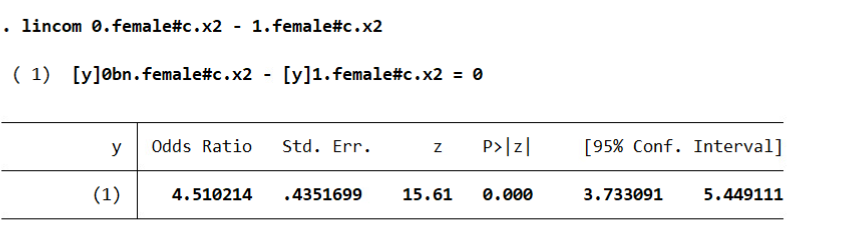And Stata defaults this to estimating a difference in the odds ratio (as far as I can tell you can’t tell Stata to do the linear coefficient after logit, would need to change the model to `glm y x, family(binomial) link(logit)` to do the linear tests).

I honestly don’t know how to really interpret this – but I have been asked for it several different times by clients. Maybe they know better than me, but I think it is more to do with people just expect to be dealing with odds ratios after a logistic regression.

So we can coerce margins to give us odds ratios:

``````* For the odds ratios
quietly margins female, at(x3=(-1(0.1)1)) expression(exp(predict(xb)))
marginsplot , yscale(log) ylabel(0.125 0.25 0.5 1 2 4 8)``````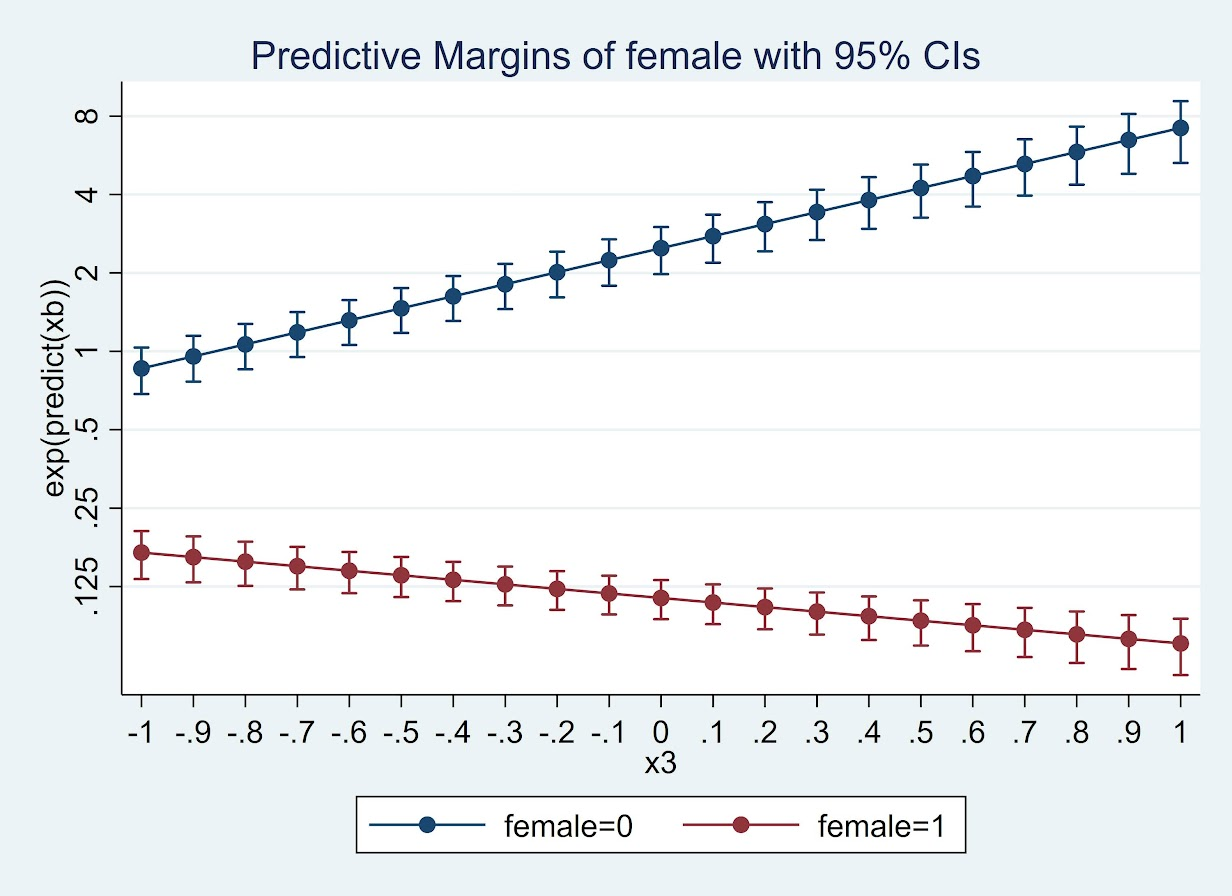Or give us the differences in the odds ratio:

``````* For the contrast in the OR
quietly margins r.female, at(x3=(-1(0.1)1)) expression(exp(predict(xb)))
marginsplot``````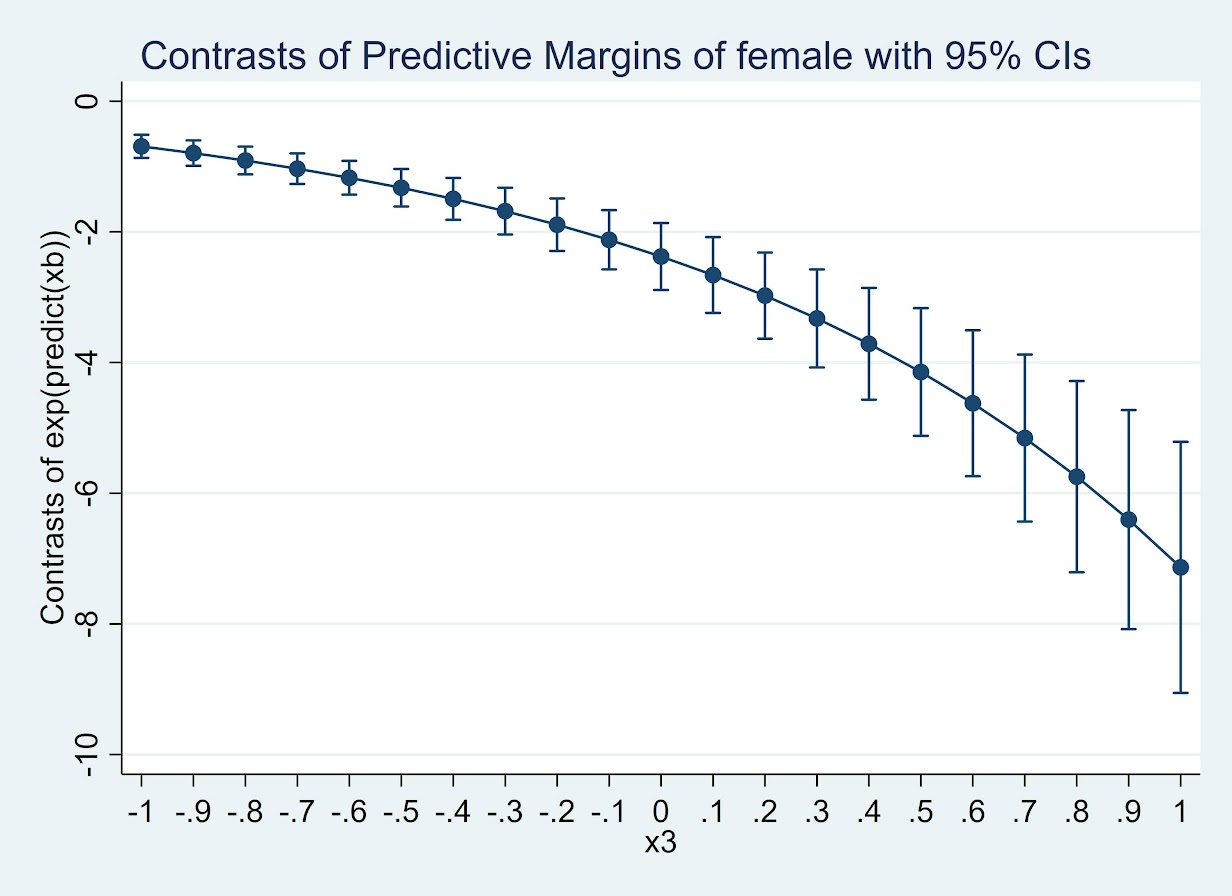(Since it is a negative number cannot be drawn on a log scale.) But again I find it much easier to wrap my head around probabilities:

``````* For the probabilities
quietly margins female, at(x3=(-2(0.1)2))
marginsplot``````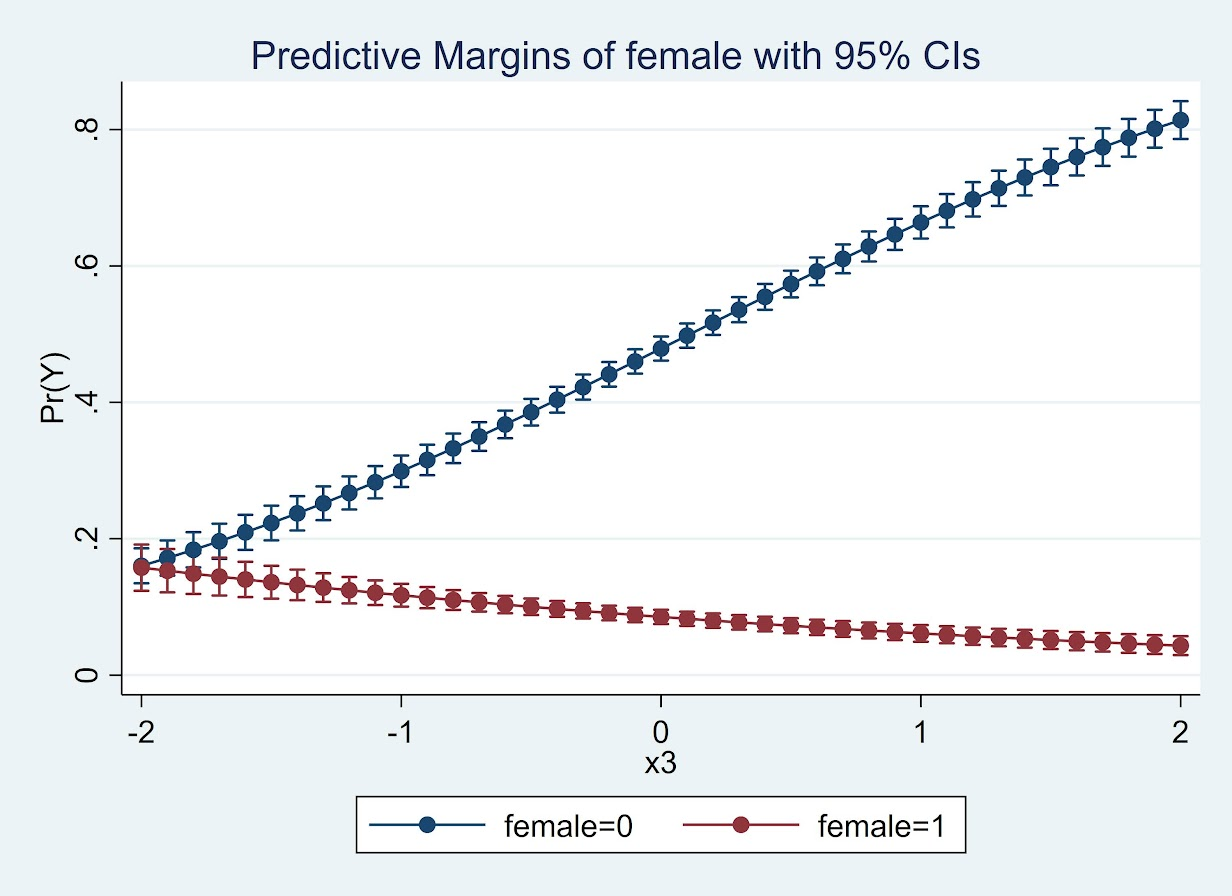So here while x3 increases, for males it increases the probability and females it decreases. The female decrease is smaller due to the smaller baseline risk in females.

So while Calli’s original question was how to do this reasonably for many different contrasts, I would prefer the actual empirical estimates of the differences. Doing a single contrast among a small number of representative values over many variables and placing in a table/graph I think is the best way to reduce the complexity.

I just don’t find the likelihood ratio tests for all equalities that informative, and for large samples they will almost always say the more flexible model is better than the restricted model.

We estimate models to actually look at the quantitative values of those estimates, not to do rote hypothesis testing.

# Making nice margin plots in Stata

For a recent working paper I had a student of mine (Jordan Riddell) help write some code to make nice margin plots in Stata, based on the work of Ben Jann and his grstyle code. Another good resource is Trenton Mize’s Sociological Science article on non-linear interactions. Here is what the end output will look like.My notes on how to get this to follow. Data and code to follow along can be downloaded from here.

## Start Up

First in my do file, I have a typical start up that sets the working directory and logs the results to a text file. I use `set more off` so I don’t have to do the annoying this and tell Stata to keep scrolling down. The next part is partly idiosyncratic to my Stata work set up — I call Stata from a centralized install location here at EPPS in UTD. I don’t have write access there, so to install commands I need to set my own place to install them on my local machine. So I add a location to `adopath` that is on my machine, and I also do `net set ado` to that same location.

Finally, for here I ssc installed `grstyle` and `palettes`. The code is currently commented out, as I only need to install it once. But it is good for others to know what extra packages they need to fully replicate your results.

``````**************************************************************************
*START UP STUFF

*Set the working directory and plain text log file
cd "C:\Users\axw161530\Dropbox\Documents\BLOG\Stata_NiceMargins\Analysis"

*log the results to a text file
log using "LogitModels.txt", text replace

*so the output just keeps going
set more off

*let stata know to search for a new location for stata plug ins

*In this script I use
*net install http://www.stata-journal.com/software/sj18-3/gr0073/
*ssc install grstyle, replace
*ssc install palettes, replace
**************************************************************************``````

## Graph Settings

Here is what I did to change my default graph settings. Again check out Ben Jann’s awesome website he made an all the great examples. That will be more productive than me commenting on every individual line.

``````**************************************************************************
*Graph Settings
grstyle clear
set scheme s2color
grstyle init
grstyle set plain, box
grstyle color background white
grstyle set color Set1
grstyle yesno draw_major_hgrid yes
grstyle yesno draw_major_ygrid yes
grstyle color major_grid gs8
grstyle linepattern major_grid dot
grstyle set legend 4, box inside
grstyle color ci_area gs12%50
**************************************************************************``````

## Data Prep

So here is pretty straight forward. I read in the data as a CSV file, generate a new variable that is the weekly average number of crimes within 1000 feet in the historical crime data (see the working paper for more details). One trick I like to use with regression models with many terms is to make a global that specifies those variables, so I don’t need to retype them a bunch. I named it `\$ContVars` here. Finally for simplicity in this script I am just examining the burglary incidents, so I get rid of the other crimes using the `keep` command.

``````**************************************************************************
*DATA PREP

*Getting the data
import delimited CrimeStrings_withData.csv

*Making the previous densities per time period
generate buff_1000_1 = buff_1000 * (7/1611)

*control variables used in the regression
global ContVars "d1 d2 d3 d4 d5 d6 d7 d8 d9 d10 d11 d12 d13 d14 d15 d16 d17 d18 whiteperc blackperc hispperc asianperc under17 propmove perpoverty perfemheadhouse perunemploy perassist i.month c.dateint"

*For here I am just examining burglary incidents
keep if crimetype == 3
**************************************************************************``````

## Making a Nice marginsplot

So basically what I want to do in the end is to draw an interaction effect between a dummy variable (whether a crime resulted in an arrest) and a continuous variable (the historical crime density at a location). I am predicting whether a crime results in a near-repeat follow up — hot spots with more crime on average will have more near-repeats simply by chance.

When displaying that interaction effect though, I only want to limit it to the support of the historical crime density in the sample. Or stated another way, the historical crime density variable basically ranges from 0 to 2.5 in the sample — I don’t care what the interaction effect is then at a historical crime density of 3.

To do that in Stata, I use `summarize` to get the min/max of that historical crime density and pipe them into a global. The Grid global will then tell Stata how often to calculate those effects. Too few and the plot may not look smooth, too many and it will take margins forever to calculate the results. Here 100 points is plenty.

``````*I will need this later to draw the margins over the support
*Of the prior crime density
summarize buff_1000_1
global MyMin = r(min)
global MyMax = r(max)
global Grid = (\$MyMax-\$MyMin)/100``````

This may seem overkill, as I could just fill in those values by hand later. If you look at my replication code for my paper though, I ended up doing this same thing for four different crimes and two different estimates, so I wanted as automated approach that avoids as many magic numbers as possible.

Now I estimate my logistic regression model, with my interaction effect and the global `\$ContVars` control variables I specified earlier. Here I am predicting whether a burglary has a follow up near-repeat crime (within 1000 feet and 7 days). I think an arrest will reduce that probability.

``````*Now estimate the logit model
logit future0_1000_7 i.arrest c.buff_1000_1 i.arrest#c.buff_1000_1 \$ContVars``````

Note that the estimate of the interaction effect looks like this:

``````--------------------------------------------------------------------------------------
future0_1000_7 |      Coef.   Std. Err.      z    P>|z|     [95% Conf. Interval]
---------------------+----------------------------------------------------------------
1.arrest |  -.0327821    .123502    -0.27   0.791    -.2748415    .2092774
buff_1000_1 |   1.457795   .0588038    24.79   0.000     1.342542    1.573048
|
arrest#c.buff_1000_1 |
1  |  -.5013652   .2742103    -1.83   0.067    -1.038807    .0360771
``````

So how exactly do I interpret this? It is very difficult to interpret the coefficients directly — it is much easier to make graphs and visualize what those effects actually mean on the probability of a near-repeat burglary occurring.

Now the good stuff. Basically I want to show the predicted probability of a near-repeat follow up crime, conditional on whether an arrest occurred, as well as the historical crime density. The first line uses `quietly`, so I don’t get the full margins table in the output. The second is just all the goodies to make my nice grey scale plot. Note I name the plot — this will be needed for later combining multiple plots.

``````*Create the two margin plots
quietly margins arrest, at(c.buff_1000_1=(\$MyMin (\$Grid) \$MyMax))
marginsplot, recast(line) noci title("Residential Burglary, Predictive Margins") xtitle("Historical Crime Density") ytitle("Pr(Future Crime = 1)") plot1opts(lcolor(black)) plot2opts(lcolor(gs6) lpattern("--")) legend(on order(1 "no arrest" 2 "arrest")) name(main)``````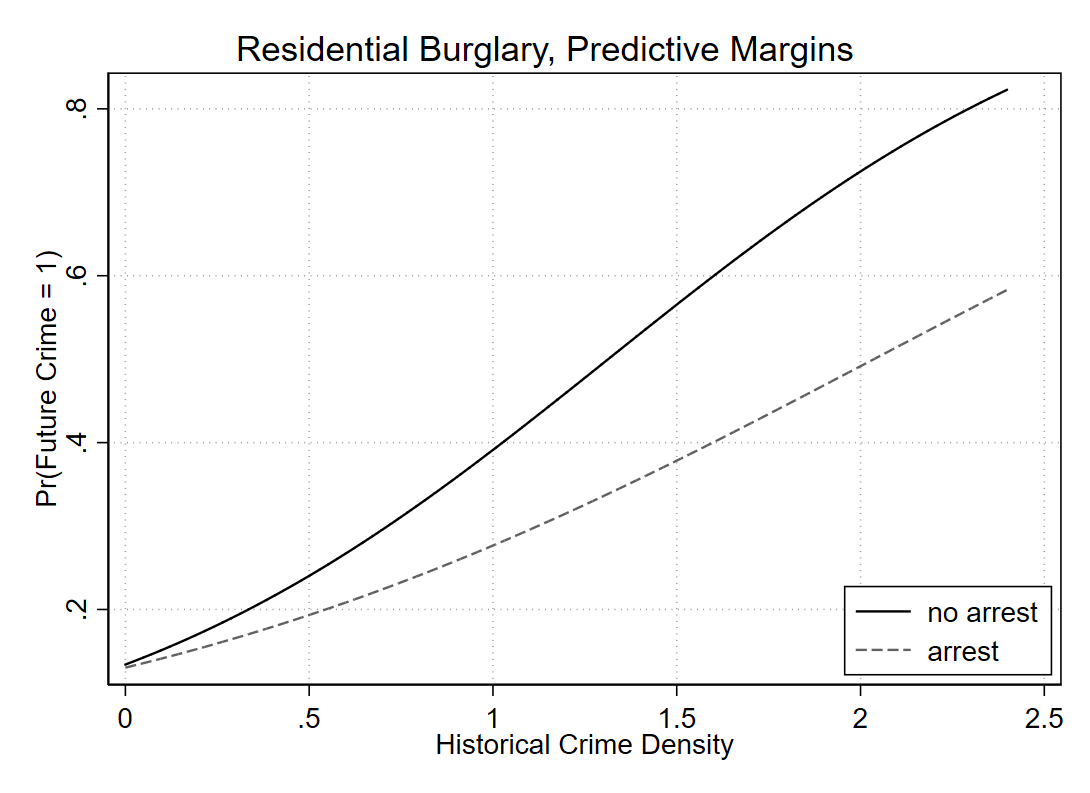You could superimpose confidence intervals on the prior plot, but those are the pointwise intervals for the probability for each individual line, they don’t directly tell you about the difference between the two lines. Viz. the difference in lines often can lead to misinterpretation (e.g. remember the Cleveland example of viz. the differences in exports/imports originally drawn by Playfair). Also superimposing multiple error bands tend to get visually messy. So a solution is to directly graph the estimate of the difference between those two probabilities in a separate graph. (Another idea though I’ve seen is here, a CI of the difference set to the midpoint of the two lines.)

``````quietly margins, dydx(arrest) at(c.buff_1000_1=(\$MyMin (\$Grid) \$MyMax))
marginsplot, recast(line) plot1opts(lcolor(gs8)) ciopt(color(black%20)) recastci(rarea) title("Residential Burglary, Average Marginal Effects of Arrest") xtitle("Historical Crime Density") ytitle("Effects on Pr(Future Crime)") name(diff)``````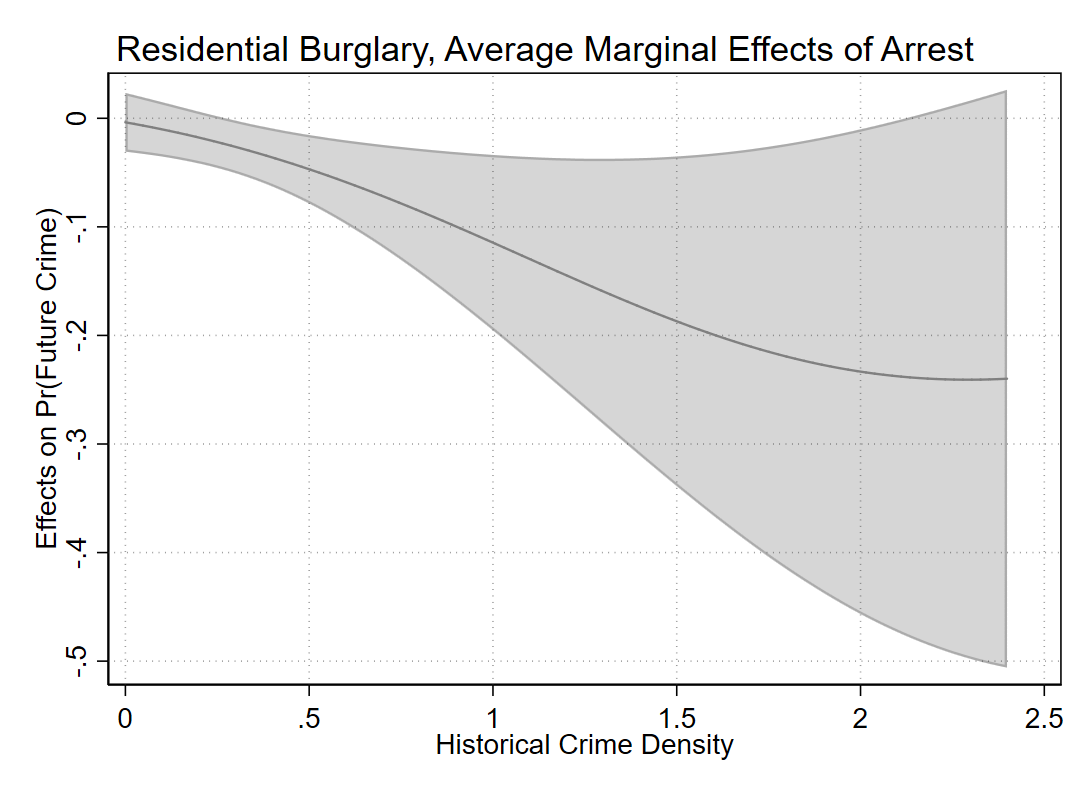Yay for the fact that Stata can now draw transparent areas. So here we can see that even though the marginal effect grows at higher prior crime densities — suggesting an arrest has a larger effect on reducing near repeats in hot spots, the confidence interval of the difference grows larger as well.

To end I combine the two plots together (same image at the beginning of the post), and then export them to a higher resolution PNG.

``````*Now combining the plots together
graph combine main diff, xsize(6.5) ysize(2.7) iscale(.8) name(comb)
graph close main diff
graph export "BurglaryMarginPlot.png", width(6000) replace``````I am often doing things interactively in the Stata shell when I am writing up scripts. Including redoing charts. To be able to redo a chart with the same name, you need to not only use `graph close`, but also `graph drop` it from memory. Then just dropping all the data and using `exit` will finish out your script and close down Stata entirely.

``````**************************************************************************
*FINISHING UP THE SCRIPT

*closing the combined graph
graph close comb

*This is necessary if you want to reuse the plot names
graph drop _all

*Finish the script.
drop _all
exit, clear
**************************************************************************  ``````